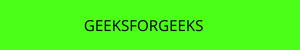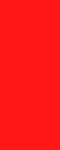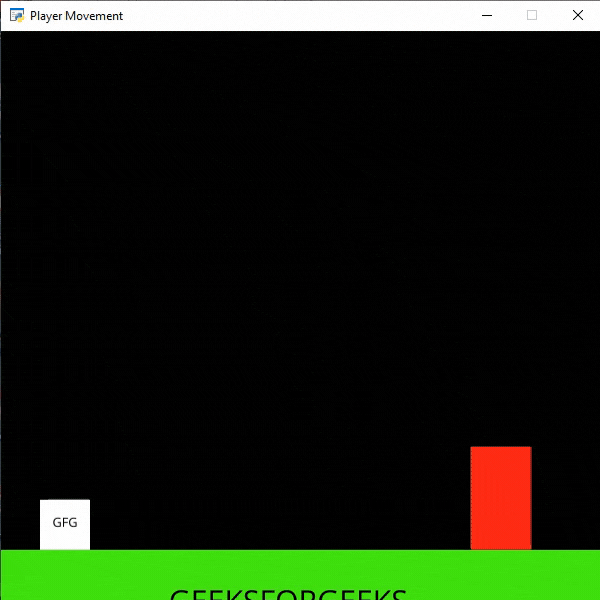GeeksforGeeks App
Open AppBrowser
Continue

## Related Articles

In this example, we are going to add one ladder on the screen which our player can climb.  For this, we will use some functions:

draw_text(): This function is used to draw text to the screen using Pyglet’s label.

Syntax: arcade.draw_text(text, x, y, color, size, width, align, font_name)

Parameters:

• text: Text we want to display
• x : x coordinate
• y : y coordinate
• color : color of the text
• size : Size of the font
• width : Width of the text
• align : Alignment of the text
• font_name : Name of the font

PhysicsEnginePlatformer(): Simplistic physics engine for use in a platformer.

Parameters:

• player_sprite: sprite of the player
• platforms: The sprites it can’t move through
• gravity: Downward acceleration per frame

Sprites Used():In the below example, we are going to create a MainGame() class. Inside this class first, we are going to initialize some variables for velocity, scene, ladder sprite, and player’s sprite then we will create 4 functions inside this class.

• on_draw(): Inside this function, we will use our scene on the screen.
• setup(): In this function, we will initialize our scene object then we will load our player and platform’s sprites then we will load our ladder sprite and initialize the starting x and y coordinate. After that, we will call the PhysicsEnginePlatformer() function. We will pass our ladder as a parameter in our PhysicsEnginePlatformer() function.
• on_update(): In this function, we will update the physics engine and the x coordinates of the player’s sprite.
• on_key_press() and on_key_release(): In this function, we will change the value of the velocity variable according to the keyboard key that is pressed or released.

## Python3

 `# Importing arcade module``import` `arcade`` ` `# Creating MainGame class``class` `MainGame(arcade.Window):``    ``def` `__init__(``self``):``        ``super``().__init__(``600``, ``600``,``                         ``title``=``"Player Movement"``)`` ` `        ``# Initializing a variable to store``        ``# the velocity of the player``        ``self``.vel_x ``=` `0`` ` `        ``# Creating variable for ladder sprite``        ``self``.ladder ``=` `None`` ` `        ``# Creating variable to store player sprite``        ``self``.player ``=` `None`` ` `        ``# Creating variable for our game engine``        ``self``.physics_engine ``=` `None`` ` `    ``# Creating on_draw() function to draw on the screen``    ``def` `on_draw(``self``):``        ``arcade.start_render()`` ` `        ``# Drawing our scene``        ``self``.scene.draw()`` ` `    ``def` `setup(``self``):``       ` `         ``# Initialize Scene object``        ``self``.scene ``=` `arcade.Scene()`` ` `        ``# Loading ladder sprite``        ``self``.ladder ``=` `arcade.Sprite(``"Ladder.png"``, ``1``)`` ` `        ``# Initializing the starting coordinates``        ``self``.ladder.center_x ``=` `500``        ``self``.ladder.center_y ``=` `110`` ` `        ``# Adding the ladder sprite in the scene``        ``self``.scene.add_sprite(``"Ladder"``, ``self``.ladder)`` ` `        ``# Creating different sprite lists``        ``self``.scene.add_sprite_list(``"Player"``)``        ``self``.scene.add_sprite_list(``"Platforms"``,``                                   ``use_spatial_hash``=``True``)`` ` `        ``# Adding player sprite``        ``self``.player_sprite ``=` `arcade.Sprite(``"Player.png"``, ``1``)`` ` `        ``# Adding coordinates for the center of the sprite``        ``self``.player_sprite.center_x ``=` `64``        ``self``.player_sprite.center_y ``=` `90`` ` `        ``# Adding Sprite in our scene``        ``self``.scene.add_sprite(``"Player"``, ``self``.player_sprite)`` ` `        ``# Adding platform sprite according to level``        ``platform ``=` `arcade.Sprite(f``"Platform.png"``, ``1``)``         ` `        ``# Adding coordinates for the center of the platform``        ``platform.center_x ``=` `300``        ``platform.center_y ``=` `32``        ``self``.scene.add_sprite(``"Platforms"``, platform)`` ` `        ``# Creating Physics engine``        ``self``.physics_engine ``=` `arcade.PhysicsEnginePlatformer(``            ``self``.player_sprite, ``self``.scene.get_sprite_list(``                ``"Platforms"``), ``0.5``, ``self``.scene.get_sprite_list(``"Ladder"``)``        ``)`` ` `    ``# Creating on_update function to``    ``# update the x coordinate``    ``def` `on_update(``self``, delta_time):`` ` `        ``# Changing x coordinate of player``        ``self``.player_sprite.center_x ``+``=` `self``.vel_x ``*` `delta_time``         ` `        ``# Updating the physics engine to move the player``        ``self``.physics_engine.update()`` ` `    ``# Creating function to change the velocity``    ``# when button is pressed``    ``def` `on_key_press(``self``, symbol, modifier):`` ` `        ``# Checking the button pressed``        ``# and changing the value of velocity``        ``if` `symbol ``=``=` `arcade.key.LEFT:``            ``self``.vel_x ``=` `-``300``        ``elif` `symbol ``=``=` `arcade.key.RIGHT:``            ``self``.vel_x ``=` `300``        ``elif` `symbol ``=``=` `arcade.key.UP:``            ``if` `self``.physics_engine.is_on_ladder():``                ``self``.player_sprite.change_y ``=` `2``            ``elif` `self``.physics_engine.can_jump():``                ``self``.player_sprite.change_y ``=` `15``        ``elif` `symbol ``=``=` `arcade.key.DOWN:``            ``if` `self``.physics_engine.is_on_ladder():``                ``self``.player_sprite.change_y ``=` `-``2`` ` `    ``# Creating function to change the velocity``    ``# when button is released``    ``def` `on_key_release(``self``, symbol, modifier):`` ` `        ``# Checking the button released``        ``# and changing the value of velocity``        ``if` `symbol ``=``=` `arcade.key.LEFT:``            ``self``.vel_x ``=` `0``        ``elif` `symbol ``=``=` `arcade.key.RIGHT:``            ``self``.vel_x ``=` `0``        ``elif` `symbol ``=``=` `arcade.key.UP ``or` `symbol ``=``=` `arcade.key.DOWN:``            ``if` `self``.physics_engine.is_on_ladder():``                ``self``.player_sprite.change_y ``=` `0`` ` ` ` `# Calling MainGame class``game ``=` `MainGame()``game.setup()``arcade.run()`

Output:My Personal Notes arrow_drop_up